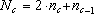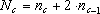## Higher Dimensions

Tiling higher dimensions works in much the same way as tiling 3-space. The same basic idea of placing truncated polytopes, (4-dimensional polyhedra), into their non-truncated lattice form and then looking for the spaces in between remains central to finding the polytopes that fill the space in between. The concepts of duality and face-to-face angle (in 4-space, cell-to-cell angle) remain germane in higher dimensions.

When figuring out the dimensional characteristics, (vertices, edges, faces, cells, etc.), of the tetrahedron, cube, and octahedron, a most amazing thing was noticed. Whilst calculating the characteristics for the tetrahedron it suddenly became apparent that the numbers were falling into the table in the same pattern numbers fall out in Pascals triangle!

Tetrahedron
 Dimensions vertices edges faces cells 4-cells 5-cells 2 3 3 1 3 4 6 4 1 4 5 10 10 5 1 5 6 15 20 16 6 1 6 7 21 35 35 21 7

Immediately we began to look at the cube and octahedron and discovered that they were exhibiting mirror imaged characteristics. In retrospect, it is obvious that the duality between these two polyhedra would produce such an effect. We were able to deduce the formula for figuring out subsequent dimensional characteristics for the two polyhedra. For the cube, a formula of, where N is the new object being calculated, n is the object one dimension below, and c is the characteristic.

Cube
 Dimensions vertices edges faces cells 4-cells 5-cells 2 4 4 1 3 8 12 6 1 4 16 32 24 8 1 5 32 80 80 40 10 1 6 64 192 240 160 60 12

The octahedron works in a mirrored way in which the equation used to calculate the dimensional characteristics is.

Octahedron
 Dimensions vertices edges faces cells 4-cells 5-cells 2 4 4 1 3 6 12 8 1 4 8 24 32 16 1 5 10 40 80 80 32 1 6 12 60 160 240 192 64

It has not escaped our attention that there is probably some other Pascal triangle like sequencing that produces these strange and curious numbers. Our current ideas rest on Pascal tetrahedrons and corners of cubes, however, no solution has yet been found.